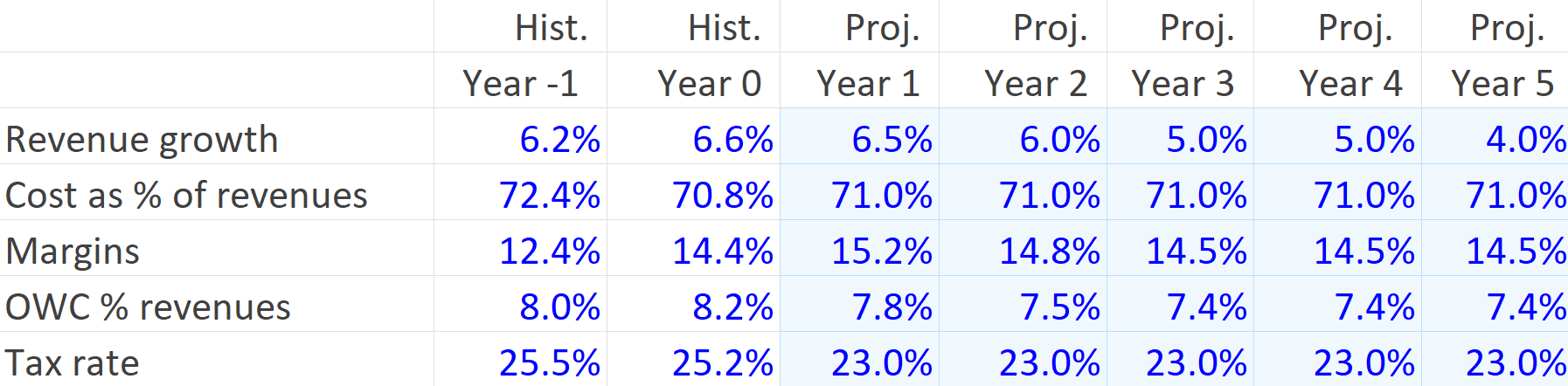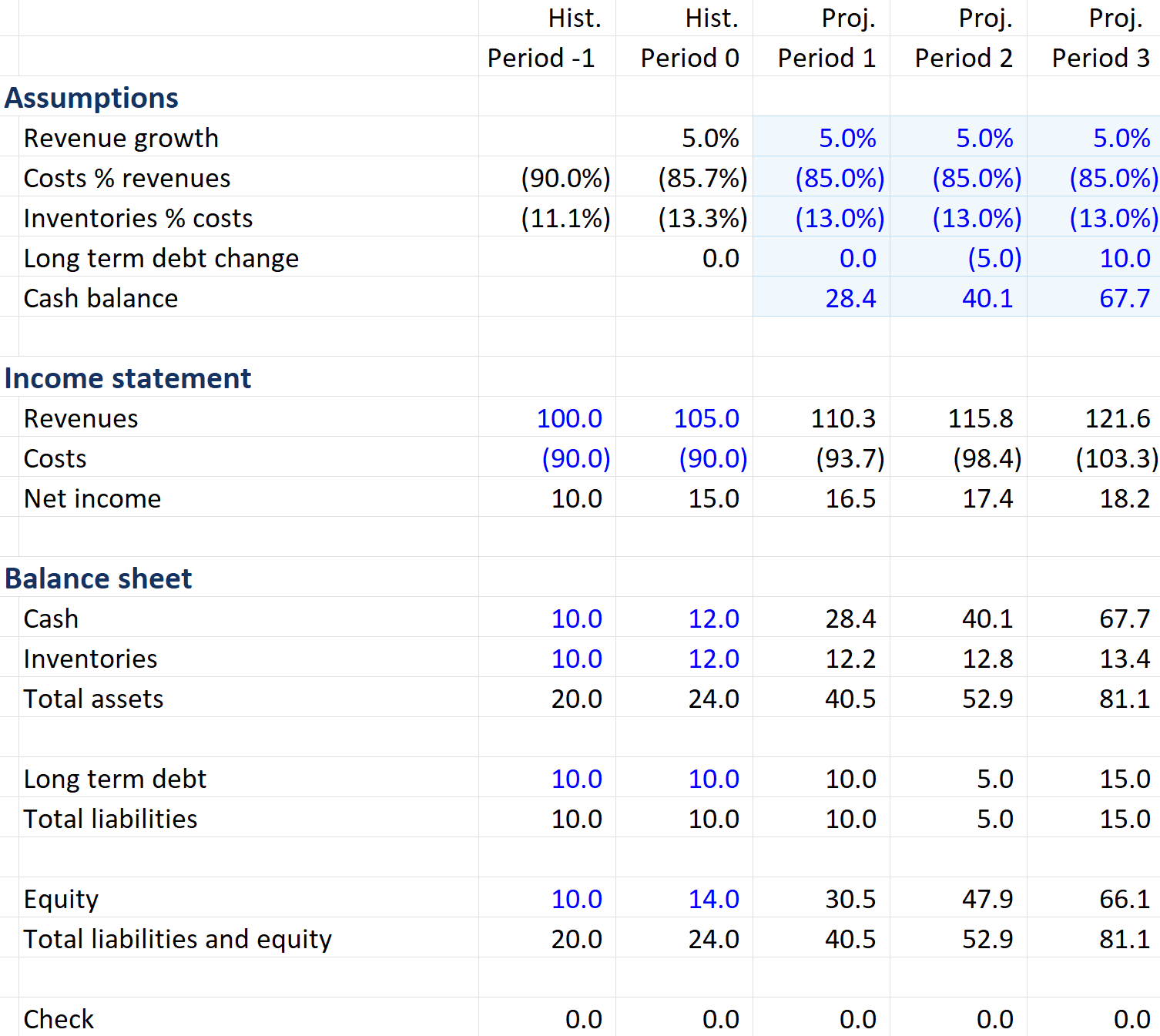## What are “Financial Modeling Assumptions”?

Financial modeling assumptions form one of the four components in the forecasting process within the realm of financial modeling – which is used to project or forecast a company’s financial performance over a specific period of time (eg. 5 years, 10 years, etc.). These assumptions play a pivotal or central role in forecasting financial data, as the forecasts are based on these assumptions. For example, if historical revenue has gone up 10% every historical year, the assumption can be for this to continue (at 10%) into the forecasting period. Therefore, based on assumptions, if year 0 (historical period) revenue was US\$100, this year’s forecast revenue would be US\$110.

The four components of the forecasting process are:

1. Inputting publicly available or private company historical data
2. Constructing ratios and statistics on this historical data
3. Creating assumptions for future performance based on the historical performance
4. Forecasting financial data based on the assumptions

The forecasting process necessitates the preparation of the company’s financial statements – balance sheet, income statement, and cash flow statements, based on financial modeling assumptions.

## Key Learning Points

• Financial modeling assumptions refer to the creation of assumptions about a company’s future growth based on analyzing its historical performance
• The set of assumptions made about future business conditions drive the forecasts of a company’s financial statements and is the basis of building a financial model
• The financial modeler has design choices to make while constructing a financial model and the forward assumptions which drive the model output

## Future Assumptions – Salient Features

Modeling assumptions dictate the forecast figures in a financial model. The assumptions will decide the forecasts of a company’s balance sheet, cash flows, and income statement, for example, forecasts of line items such as revenues and costs.

Further, financial modeling assumptions such as earnings and cash flows must be forecast carefully into the future, as the outlook for these items are important determinants of share price.

## Where Do Assumptions Come From?

So, how do we come up with assumptions figures? Historical figures are an important first step. If we see the historical sales going up by 5% in every historical period, we may assume that the future sales in the forecast period will also go up by 5%. If last year’s sales were 100, forward sales would be 105. Our forecast assumption would be 5%. However, assumptions can include sales growing faster or slower than the previous year.

The other place to look for future assumptions is within industry research in case there are any factors that may have a positive or negative impact on this company. Our initial 5% assumption for the future sales growth may be increased to reflect strong economic conditions.

## Assumption Design Choices

The financial modeler has several design choices to make when constructing a model – how many excel sheets to use, where to put the model assumptions, and how to format the numbers.

The first design choice is where to put the assumptions figures. The first option is to put all of the assumptions in one place in the model i.e. they are ‘blocked’ together. This would mean that the assumptions regarding revenue growth, operating costs, taxes rate, etc are all together. Blocked assumptions are important in large models – and often mean having them all in one tab.

A second option is to have the assumptions spread throughout the output or model. These are called “threaded” assumptions. For example, if you have the revenue growth assumption (say 3%), you would then have the output revenue in the row above or below the assumption – so you have the driver (3%) and the output (forecast revenue) together. The benefit here is that having both together means that we can see what is driving the output, and can spot errors more easily.

Financial modeling assumptions should always be explicitly documented in a model so that a user can see them easily and then change them when required. It is best modeling practice to put the assumption in its own cell, which is then linked to when building the forecast data. This means that the output can be changed simply by amending the content of the assumption cell (for example sales growth changing from 4% to 5%). It is never appropriate to embed the assumption in the output formula. Doing this means that the driver is invisible and cannot be changed without adjusting formulas.

## Formatting Assumptions

Now that the assumptions are hard-coded in their own cell, they need to be formatted as assumptions. The font color is changed from black to blue, and the background fill color is changed from white to pale blue. See the screenshots below.

Assumptions are formatted differently to historical numbers. Historical numbers are facts and are formatted with just a blue font, no background color. Whereas assumptions are only estimates which may change – they are facts. These have a blue font with a pale blue background.

## Assumptions Placed Near Historical Numbers

Many financial modelers will position the forecast assumption and the equivalent historical ratio next to each other, so that it is easy to see how the ratio changes over time, and so the forecast assumptions can be seen to be in line with the historical number. This is a sense check and is an important part of building an error free model. Any variation in this trend should be adequately explained with a well-documented comment.

## Historical Ratios and Forecast Assumptions – Generating Assumptions From Historical Data Example

Below, we see two years of actual historical data and 5 years of estimated forecast assumptions in Year 1 – Year 5. We can see that in the historical period for Year -1 and Year 0, annual revenue growth was 6.2% and 6.6% respectively. Looking at this historical data helps us to understand the business in the past and consider where it is likely going in the future. As revenue has grown by 6.2% and 6.6% in the past, the modeler may think it reasonable to assume growth of 6.5% in the first projected year, and then slightly lower growth of 5% and 4% after that.

We can use the historical figures to come up with the forecast assumptions as well as using additional research for this purpose such as information from the company’s management or our own opinion. The revenue growth assumptions are laid out below. These forward assumptions will drive the forecast model for 5 years into the future. Notice how the formatting of the assumptions in the projected years is different to the historical hard-coded numbers.## Forecast Model – Income Statement and Balance Sheet

In the free download is a template file where you can build an income statement and balance sheet using assumptions.  Historical figures are also given, from which historical ratios and statistics are calculated. These historical ratios help in coming up with the forecast assumptions in forecast periods 1 to 3.

The example below shows the template file. Notice the formatting, and how it is slightly different to the previous example above. Below, the historical ratios are not hard-coded inputs. Instead they are calculated from the historical numbers. So the historical ratios have a black font color to indicate they are calculations, and instead it is the historical figures e.g. Revenues, that are blue font as they are the hard-coded numbers in this model. The assumptions remain the same as the previous example, with blue font and blue background to indicate they are hard-coded estimates rather than historical facts.

For the forecast process, we first have the historical data, then ratios calculated using the historical data, which gives us suggested numbers for the forecast assumptions and ultimately our financial forecasts.

Other steps to do at the beginning of a model include calculating historical subtotals. Calculations are needed for:

• Historical net income (revenues minus costs)
• Historical total assets (cash + inventories)
• Historical total liabilities, and total liabilities plus equity
• A balance check to ensure that total liabilities + equity – total assets = 0

Notice in the example below that the subtotals are black font color, to indicate they are calculations rather than hard-coded numbers.

With the historical subtotal done, next we move onto the forecast period, using the assumptions. To calculate the revenues for the forecast period we will grow the last historical year’s revenue with the revenue growth assumption (5%). For costs, the assumption is 85% of revenues (i.e. 85% of US\$110.3 revenue). We can then calculate the net income for the forecast period.

Moving down to the balance sheet, the cash assumption is already given. For the calculation of inventories for the forecast period, we use the inventories as a % of costs (13%) assumption and multiply it by the costs in the forecast year (period 1 to 3). Thereafter, adding cash and inventories, we get the total assets for the projected years.

To arrive at total liabilities, we compute long-term debt for the forecast period, using the long-term debt change assumption (0%) i.e. no change from last year in the first projected year. There is no specific assumption for equity. So, we take the net income from the income statement and add it to last year’s equity (i.e. US\$16.5 + US\$14 = US\$30.5) which assumes that all net income is retained by the company, and not paid out as a dividend. We see that the balance sheet balances.

Learn how to build financial models with our financial modeling course – The Modeler.

## Free Model Template

Click the free download button to get this free Excel model template.